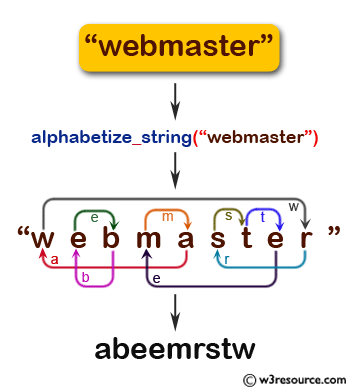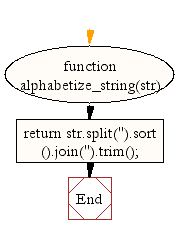# JavaScript: Alphabetize a given string

## JavaScript String: Exercise-25 with Solution

Write a JavaScript function to alphabetize a given string.

Alphabetize string : An individual string can be alphabetized. This rearranges the letters so they are sorted A to Z.
Test Data:
console.log(alphabetize_string('United States'));
Output:

Pictorial Presentation:Sample Solution:-

HTML Code:

``````<!DOCTYPE html>
<html>
<meta charset="utf-8">
<title>Write a JavaScript function to alphabetize a given string.</title>
<body>

</body>
</html>
```
```

JavaScript Code:

``````function alphabetize_string(str)
{

return str.split('').sort().join('').trim();

}
console.log(alphabetize_string('United States'));
```
```

Sample Output:

```SUadeeinsttt
```

Flowchart:Live Demo:

See the Pen JavaScript Alphabetize a given string - string-ex-25 by w3resource (@w3resource) on CodePen.

What is the difficulty level of this exercise?

﻿

## JavaScript: Tips of the Day

JavaScript: eval function

```const sum = eval('10*10+5');
```

eval evaluates codes that's passed as a string. If it's an expression, like in this case, it evaluates the expression. The expression is 10 * 10 + 5. This returns the number 105.

Ref: https://bit.ly/323Y0P6